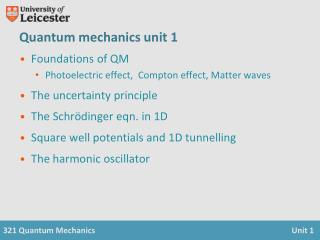DownloadDownload PresentationQuantum mechanics unit 1

# Quantum mechanics unit 1

Télécharger la présentation## Quantum mechanics unit 1

- - - - - - - - - - - - - - - - - - - - - - - - - - - E N D - - - - - - - - - - - - - - - - - - - - - - - - - - -
##### Presentation Transcript

1. Quantum mechanics unit 1 • Foundations of QM • Photoelectric effect, Compton effect, Matter waves • The uncertainty principle • The Schrödinger eqn. in 1D • Square well potentials and 1D tunnelling • The harmonic oscillator

2. Last time • Photons • Photoelectric effect • Compton effect • Matter waves • de Broglie relations • Electron diffraction • Waves related to probability www.le.ac.uk/physics -> people -> Mervyn Roy

3. Heisenberg uncertainty principle • Fundamental property of quantum systems • Course 3210 Unit 3, Rae Chapter 4 • Inherent uncertainties in predictions – or average spread of a set of repeated measurements Heisenberg - formulation of QM 1927, Nobel prize 1932

4. Example • The position of a proton is known to within 10-11 m. Calculate the subsequent uncertainty in its position 1 second later. km

5. Example • An atom in an excited state emits a photon of characteristic frequency, n. The average time between excitation and emission is 10-8 s. Calculate the irreducible linewidth of the transition. Hz

6. Schrödinger equation • Developed by induction • Tested against experiment for over 80 years • is the wavefunction. This is essentially complex – cannot be identified with any one physical property of the system Schrödinger - formulation of QM 1925-26, Nobel prize 1933

7. Schrödinger equation • related to probability dx • Wavefunction must be normalised = 1

8. Time independent Schrödinger equation eigenfunction eigenvalue

9. Constraints • The wavefunction and its first derivative must be: • Single valued • Finite • Continuous